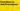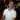# VBA Functions vs Sub Procedures

June 15, 2021

Since we’ve already discussed Sub Procedures and Functions, let’s talk about when you should use one versus the other.

There are a lot of things that you can do in either a function or a sub procedure, but some things must to be done through one or the other. Let’s go over those first.

## Sub Procedures Only

The following items can only be done with a sub procedure. It helps to keep this in mind when writing VBA code so you can avoid running into issues later on.

### Macros

All macros need an entry point and that is always going to be a sub procedure. When you record a macro, it creates a sub procedure for you, like so:

``````Sub MyRecordedMacro()
'
' MyRecordedMacro Macro
'

'
Range("A1:C1").Select
Selection.Merge
End Sub``````

If you were to change `Sub` to `Public Function`, the code itself will still work if you called it from another sub procedure, but it will not show up in the macros list in Excel.

### Events

All Events have to be done through a Sub Procedure. For example, if we want to know when a cell was selected:

``````Private Sub Worksheet_SelectionChange(ByVal target As Range)
End Sub``````

This also goes for Workbook events, UserForm events, etc.## Functions Only

### User-Defined Functions

These are functions that you would use from a worksheet cell. For example:

``````Public Function Halve(ByVal amount As Single) As Single
Halve = amount / 2
End Function``````

If you entered this into `A1`:

``=Halve(30)``

If will output `15`. You can pass in a range, too. If you entered this into `B1`:

``=Halve(A1)``

It will return `7.5`.

If you tried to change `Public Function` to `Public Sub`, you would not be able to execute the code from a cell.

## When to Choose a Function vs a Sub Procedure

Now let’s go over when you have the choice to use either a function or a sub procedure. While you can use either one for a lot of tasks, there is still one big restriction: a sub procedure cannot return a value. This is very important to remember because you can use a function for an expression, meaning that you can use it in an `If` statement and you can use it on the right-hand side of the equal `=` sign (more on these in a bit).

The following suggestions are just my opinion. If you have different reasons to use one over the other, please let me know in the comments.

### Changing Workbook Contents? Use Sub Procedures

When making changes to the workbook, like adding / deleting worksheets, changing cell values, changing cell formats, adding charts, etc., use a Sub Procedure.

In this example, we take the currently selected cell, halve it, and check if it’s less than `1`. If so, color the cell red.

``````Public Sub HalveSelection()
Dim cell As Excel.Range
Set cell = Selection

cell.Value = Halve(cell.Value)

If (cell.Value < 1) Then
cell.Interior.Color = vbRed
End If
End Sub

Public Function Halve(ByVal amount As Single) As Single
Halve = amount / 2
End Function``````

While you technically can do the same thing like change a cell’s color in a function call, I wouldn’t recommend it. To me, functions are really meant for calculating values and evaluating expressions.

### Calculating Values? Use Functions

Need to check what the budget should be? Use a function. Looking to see if a cell uses a formula? Use a function.

Using functions for calculations and expressions is very powerful. You can make your code a lot more readable when you change an expression into a function call. For example:

``````Public Sub DoubleSelection()
Dim cell As Excel.Range
Set cell = Selection

If (cell.Parent.ProtectContents = False  _
Or (cell.Parent.ProtectContents = True And cell.Locked = False)) Then
cell.Value = cell.Value * 2
Else
Debug.Print "Cannot edit cell."
End If
End Sub``````

Not too terrible to look at, but that `If` statement is a little confusing. We can make this more readable by using function names to make the intent clear:

``````Public Sub DoubleSelection()
Dim cell As Excel.Range
Set cell = Selection

If (IsEditable(cell)) Then
cell.Value = cell.Value * 2
Else
Debug.Print "Cannot edit cell."
End If
End Sub

Private Function IsEditable(ByVal cell As Excel.Range) As Boolean
IsEditable = IsWorksheetProtected(cell) = False _
Or (IsWorksheetProtected(cell) And cell.Locked = False)
End Function

Private Function IsWorksheetProtected(ByVal cell As Excel.Range) As Boolean
IsWorksheetProtected = cell.Parent.ProtectContents
End Function``````

Notice what we did here? We were able to use a custom function inside an `If` statement to determine if an expression returned `True` or `False`. You cannot do that with a sub procedure.

The other benefit to this approach is that your functions are reusable from other functions and sub procedures throughout your code.

Another thing you can do with a function that you can’t do with a sub procedure is put it on the right-hand side of an assignment.

Let’s update the line where we set the cell’s value to use a function call instead:

``````Public Sub DoubleSelection()
Dim cell As Excel.Range
Set cell = Selection

If (IsCellEditable(cell)) Then
cell.Value = DoubleAmount(cell.Value) ' <-- updated this line
Else
Debug.Print "Cannot edit cell."
End If
End Sub

Private Function IsEditable(ByVal cell As Excel.Range) As Boolean
IsEditable = IsWorksheetProtected(cell) = False _
Or (IsWorksheetProtected(cell) And cell.Locked = False)
End Function

Private Function IsWorksheetProtected(ByVal cell As Excel.Range) As Boolean
IsWorksheetProtected = cell.Parent.ProtectContents
End Function

Private Function DoubleAmount(ByVal amount As Single) As Single
DoubleAmount = amount * 2
End Function``````

Using functions this way gives you a lot of options for setting up your VBA code and making your code clean.

Wow, you read the whole article! You know, people who make it this far are true learners. And clearly, you value learning. Would you like to learn more about Excel? Please consider supporting me by buying me a coffee (it takes a lot of coffee to write these articles!).Written by Joseph who loves teaching about Excel.